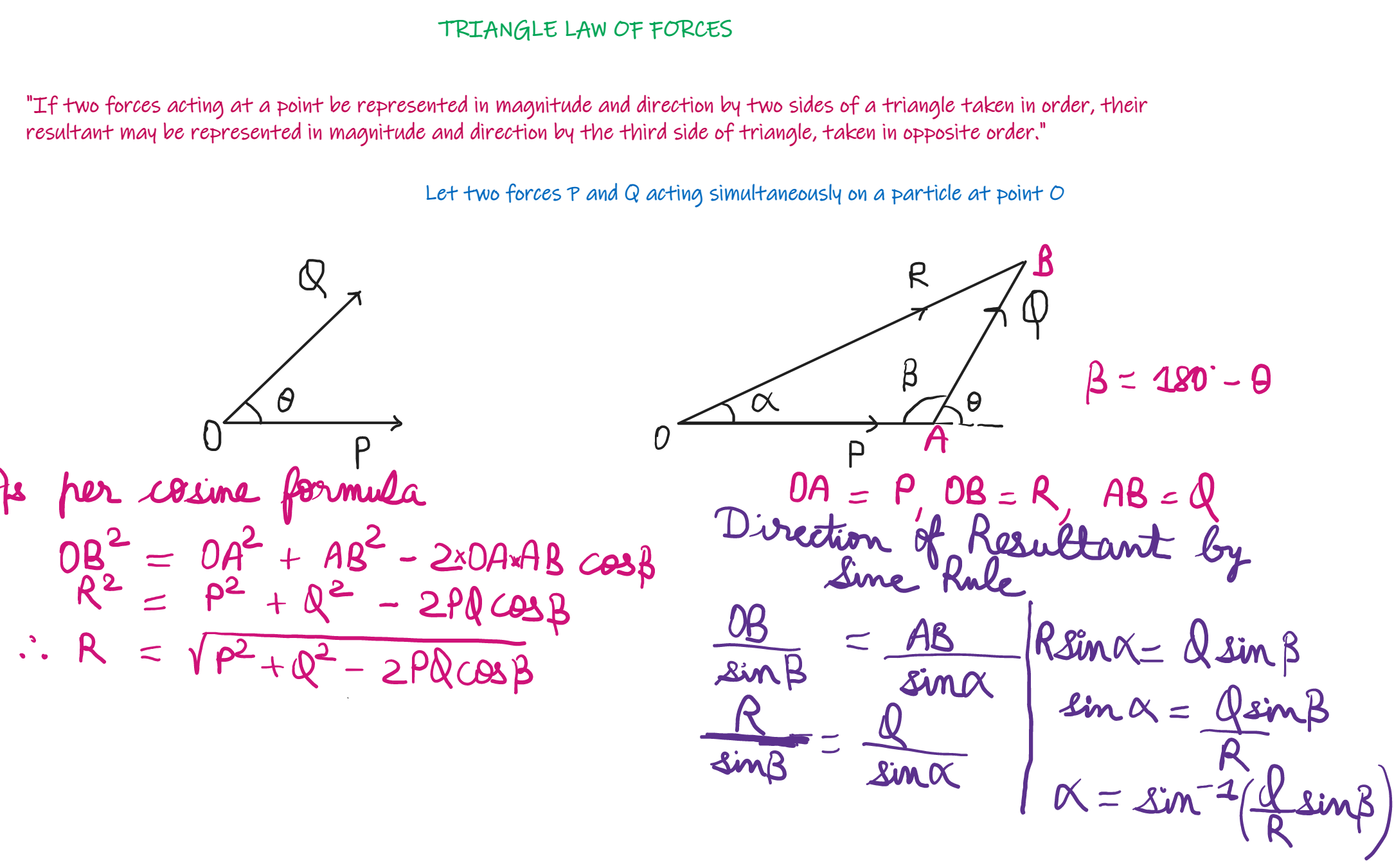# Triangle Law of Forces

“If two forces acting at a point be represented in magnitude and direction by two sides of a triangle taken in order, their resultant may br represented in magnitude and direction by the third side of a triangle taken in opposite order.”Triangle Law of Forces

Example: Two tensile forces of 20KN and 30KN are acting at a point with an angle of 60 degree between them. Find the magnitude and direction of the resultant force.